# Automatic Navigation Mesh Generation in Configuration Space

Automatic Navigation Mesh Generation in Configuration Space

By Stuart Golodetz

Walkable 3D environments can be automatically navigated. Stuart Golodetz demonstrates how navigation meshes achieve this.

The representation of the walkable area of a 3D environment in such a way as to facilitate successful navigation by intelligent agents is an important problem in the computer games and artificial intelligence fields, and it has been extensively studied. As surveyed by Tozour [ Tozour04 ], there are a variety of common ways to represent such an environment, including:

• Regular Grids . These support random-access lookup but do not translate easily into a 3D context. They also use a lot of memory and can yield aesthetically unpleasing paths for navigating agents.
• Waypoint Graphs . These connect large numbers of nodes (often manually placed) using edges that imply walkability in the game world. They were previously popular in games but are costly to build and tend to constrain agents to walking ‘on rails’ between connected waypoints.
• Navigation Meshes . These represent the walkable surface of a world explicitly using a polygonal mesh. Polygons within a navigation mesh are connected using links that imply the ability of the agent to walk/step/jump/etc. between them (see Figure 1).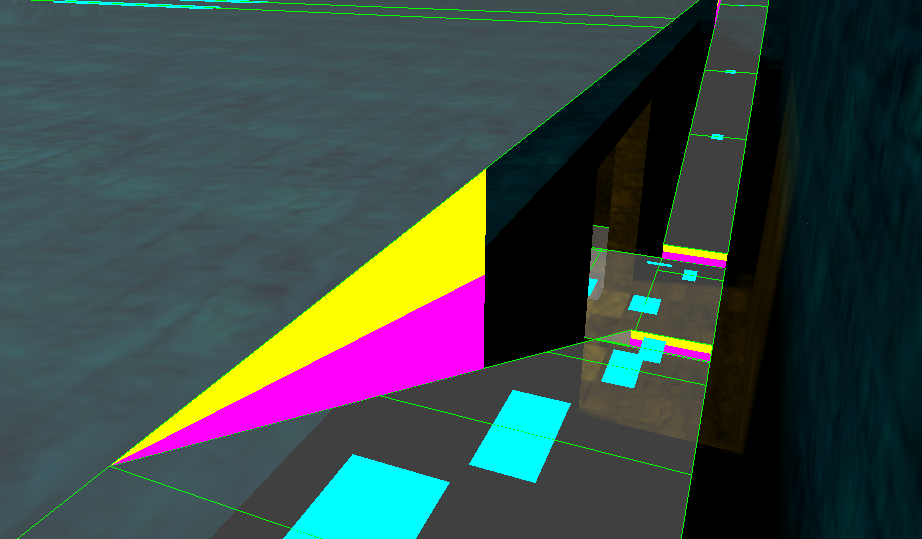An example navigation mesh and its links: cyan = walk link; magenta = step up link; yellow = step down link. (Note that in greyscale, the walk links are light grey, the step up links are dark grey and the step down links are white.) Figure 1

Since their introduction by Greg Snook [ Snook00 ], navigation meshes have proved to be a particularly successful approach due to their ability to represent the free space available around paths through the world (this is extremely useful because it provides the pathfinder with the information it needs to successfully avoid local obstacles). As a result, they have seen widespread use in both games themselves, and popular games engines such as Source and Unreal, and many games authors have contributed to their theoretical development (most notably in the Game Programming Gems and AI Game Programming Wisdom book series). There has also been significant interest from researchers in academia (e.g. see [ Hale09 , Kallmann10 , Pettré05 , VanToll11 ]).

One facet of using navigation meshes is how to build them in the first place, and numerous methods have been described in the literature. An early approach due to Tozour [ Tozour02 ] works by first determining the walkable polygons in a 3D environment by comparing their normals 1 with the up vector, and then iteratively merging together as many polygons as possible using the Hertel-Mehlhorn algorithm [ Hertel83 , ORourke94 ] and a 3 to 2 merging technique. Hamm [ Hamm08 ] generates a navigation mesh using an empirical method that involves sampling the environment to create a grid of points, identifying a subset of points both on the boundary of and within the environment, and connecting these points to form a mesh. Ratcliff [ Ratcliff08 ] creates a navigation mesh by tessellating all walkable surfaces in the world, merging the results together to form suitable nodes and then computing links between neighbouring nodes. Van Toll et al. [ VanToll11 ] build a navigation mesh for a multi-layer environment by constructing a mesh based on the medial axis for each layer and then connecting the medial axes by ‘opening’ the connections between the layers. The same authors also demonstrate how such a mesh can be dynamically modified [ VanToll12 ]. Mononen’s open-source Recast library [ Mononen09 ] first voxelizes 2 the 3D environment before running a watershed transform [ Beucher90 , Gonzalez02 ] on the walkable voxels and creating a mesh from the resulting partition of the walkable surface.

In this article, I describe the implementation of navigation mesh construction in my homemade hesperus engine [ hesperus ], based heavily on the techniques of van Waveren in [ VanWaveren01 ]. The goal is to provide a helpful, implementation-focused introduction for those with no prior experience in the area. At a high-level, the method is as follows:

• Firstly, given a 3D environment made up of brushes (simple convex polyhedra, each consisting of a set of polygonal faces), and a set of axis-aligned bounding boxes (AABBs) used to represent the possible sizes of the agents that will navigate the environment, expand the brushes by appropriate amounts (see the ‘Configuration Space’ section) to create a set of expanded brushes for each AABB.
• Next, using constructive solid geometry (CSG) techniques, union the expanded brushes for each AABB together to form a polygonal environment. Filter the polygons of the environment to find those that are walkable (judged by comparing their face normals with the up vector). This gives us the polygons of a navigation mesh for each AABB, but without any links to indicate how agents should navigate between them. See the ‘Basic Mesh Generation’ section.
• Finally, generate walk and step links between the polygons of each navigation mesh (see the ‘Walk and Step Links’ section) – these indicate, respectively, that an agent can walk from one polygon of the mesh to another, or step up/down from one polygon to another. These links can be used to generate a graph for the purposes of path planning.

The following sections look at each of these steps in more detail.

## Configuration space

When planning the movement of intelligent agents (e.g. robots), a configuration space is the space of possible configurations in which an agent can validly exist. As a first example of what this concept means and when it can be useful, consider detecting the first point of collision between a plane and an AABB that is moving by translation only – this might normally involve determining which vertex of the AABB is nearest to the plane and finding the point at which a half-ray oriented in the AABB’s direction of movement and starting at that vertex would intersect the plane (see Figure 2(a)). The configuration space alternative is to initially expand the plane in the direction of its normal by a fixed amount so that the centre of the AABB touches the expanded plane precisely when the nearest vertex of the AABB touches the non-expanded plane (see Figure 2(b)). The first point of intersection can then be calculated by finding the point at which a half-ray starting at the centre of the AABB would touch the expanded plane (see Figure 2(c)) – there is no longer a need to first determine which vertex is nearest to the plane. Put another way: by expanding the plane, we have created the space of possible configurations for the centre of the AABB, and thereby restricted our testing to making sure that the AABB’s centre is always in a valid location.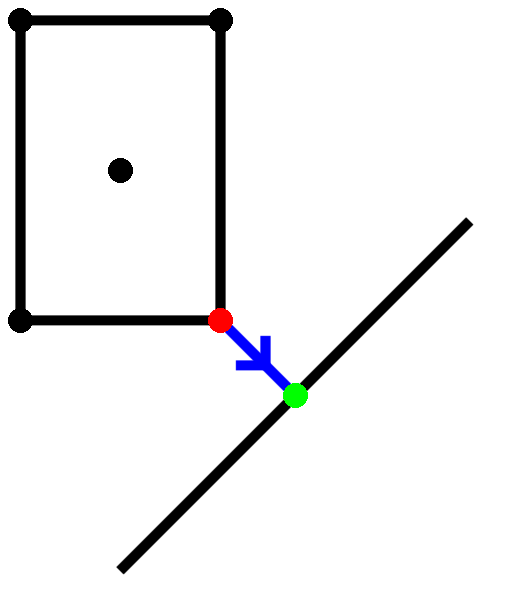(a) Finding the intersection of a half-ray through the nearest vertex of the AABB with the plane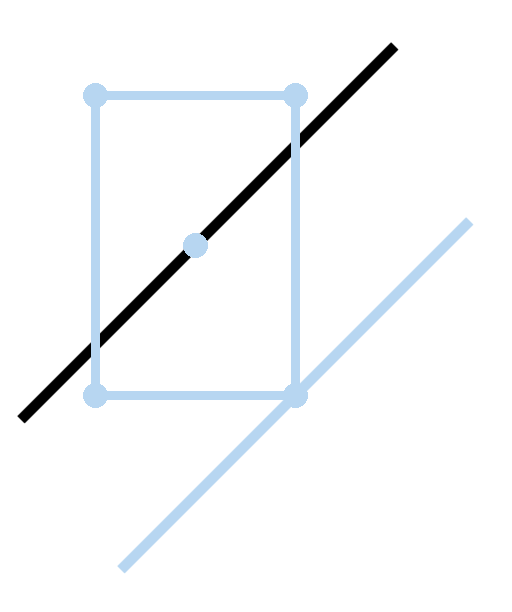(b) Expanding the plane to form a configuration space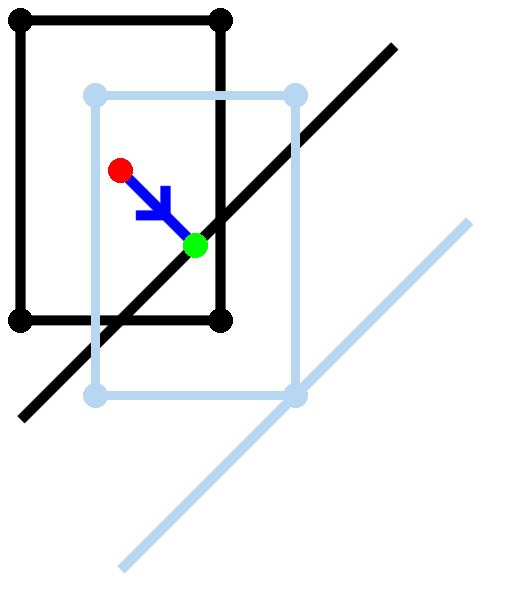(c) Finding the intersection of a half-ray through the centre of the AABB with the expanded plane Figure 2

As explained in [ VanWaveren01 ], the concept of configuration space can be extended to an entire 3D environment, allowing us to test an agent represented as an AABB against such an environment using only point-based (rather than AABB-based) intersection tests: this was the approach taken in the popular Quake III Arena game. Starting with a brush-based 3D environment (i.e. one that is built up by combining instances of simple convex polyhedra such as cuboids, cylinders and cones – a common approach in 3D world editors), a configuration space for agents with a specific AABB can be constructed by expanding each brush by an appropriate amount (see Figures 3(a) and 3(b)). Note that expanding each brush correctly can require the introduction of additional bevel planes as described in [ VanWaveren01 ] (see Figure 3(c)).

 A configuration space can be generated for the entirety of a brush-based environment by expanding all of the brushes by an appropriate amount: (a) shows a brush-based environment, together with the range of movement of a simple agent; (b) shows the configuration space that would be generated for the centre of that agent; (c) shows that expanding non-axis-aligned brushes may require bevel planes (shown in green) in order to correctly determine the range of movement.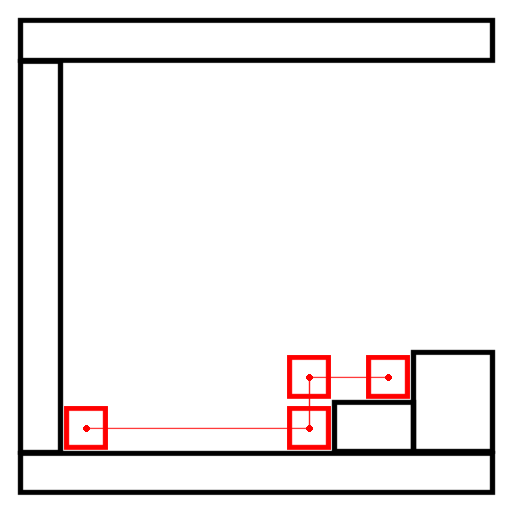(a) A brush-based environment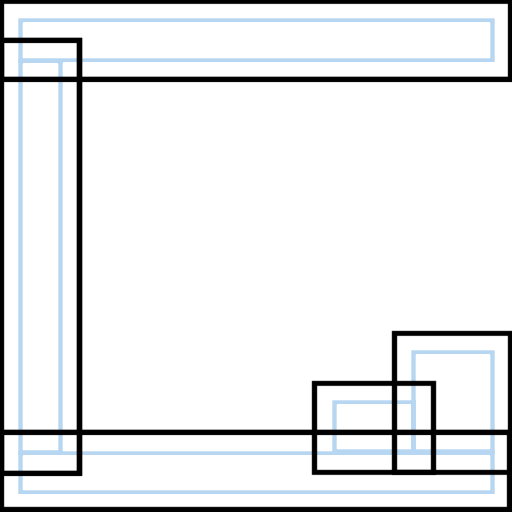(b) Configuration space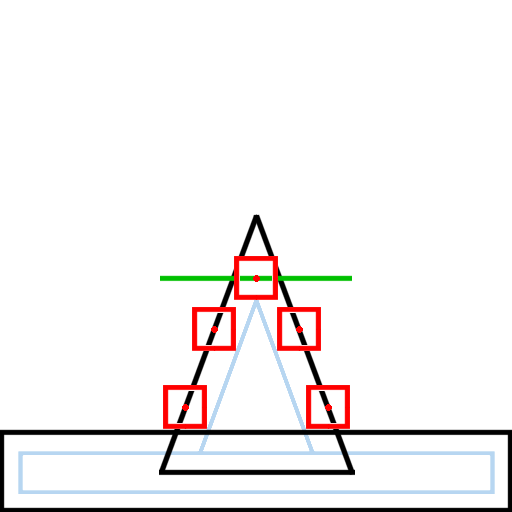(c) A bevel plane Figure 3

## Basic mesh generation

### Brush unioning

Having expanded the brushes of a brush-based environment to construct a configuration space for an AABB in the manner described, the next step is to union the expanded brushes together to generate a set of polygons that represent the expanded environment as a whole. These polygons can then be processed further to construct a navigation mesh.

In conceptual terms, the process of brush unioning is relatively simple: given an input set of brushes, each of which consists of a set of (outward-facing) polygonal faces, it suffices to clip each brush face to all the other brushes within range of its own brush in the environment. From an implementation perspective, a convenient way to do this is to build a binary space partitioning (BSP) tree for each brush and clip each face to the trees of the other brushes. The high-level implementation of this process can be found in Listing 1 and full source code is available online [ hesperus ]. The end result is a set of polygons that represent the expanded environment.

 ```function union_all : (brushes: Vector) -> List var result: List; // Build a tree for each brush. var trees: Vector := map(build_tree, brushes); // Determine which brushes can interact. var brushesInteract: Vector>; for each bi, bj in brushes if j = i then brushesInteract(i, j) := false; else brushesInteract(i, j) := in_range(bi, bj); // Clip each polygon to the tree of each brush // within range of its own brush. for each bi in brushes for each f in faces(bi) var fs: List := [f]; for each bj in brushes if brushesInteract(i, j) then fs := clip_polygons(fs, trees(j), i < j); result.splice(result.end(), fs); return result; ``` Listing 1

### Finding walkable polygons

Given the set of polygons generated in the previous section, finding those polygons that are walkable is straightforward: it suffices to compare the angle between each polygon’s normal,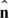, and the up vector (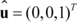) to some predefined threshold. The angle can be computed using the dot product: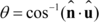We then keep precisely those polygons whose angle is less than or equal to the threshold. In the hesperus engine, a suitable threshold for human characters was found to be π/4 (i.e. 45 degrees to the horizontal).

To generate simple links between walkable polygons in a navigation mesh, the general strategy is as follows:

• We first create an edge plane table that maps each vertical plane through one or more walkable polygon edges to two sets of edges that lie in the plane (edges in one set are oriented in the same direction as a ‘canonical’ plane; edges in the other have the opposite orientation).
• For each plane in the table and for each ordered pair of opposing edges for that plane, we check to see whether any links need to be created. This is done by transforming the opposing edges into a 2D coordinate system in the plane and calculating the intervals (if any) in which the various types of link need to be created.

### Edge plane table construction

The edge plane table is a map of type Plane to ({Edge},{Edge}). To construct it, we proceed as shown in Listing 2.

 ```function build_edge_plane_table : (walkablePolygons: List<Polygon>) -> Map<Plane,EdgeRefsPair,PlaneOrdering> var ept: Map<Plane,EdgeRefsPair,PlaneOrdering>; for each poly in walkablePolygons for each p1 in vertices(poly) var p2: Vec3 := next_vertex(poly,p1); var plane: Plane := make_vertical_plane(p1,p2); var canon: Plane := plane.make_canonical(); var sameFacing: bool := plane.normal().dot(canon.normal()) > 0; if sameFacing then ept(canon).sameFacing.add(EdgeRef(poly,p1)); else ept(canon).oppFacing.add(EdgeRef(poly,p1)); return ept; ``` Listing 2

For each edge of a walkable polygon, we first build the vertical plane passing through it and then add it to the table based on its facing with regard to the ‘canonical’ vertical plane through the edge. This has the effect of separating the edges of walkable polygons into two sets on each vertical plane. These can then be checked against each other in a pairwise manner to create navigation links – see Figure 4 for an example.

An illustration of (part of) the edge plane table for the hesperus test level called Ramp: the ordered pairs of opposing edges are (e1,e3), (e2,e3), (e3,e1), (e3,e2), (e4,e5) and (e5,e4). The first four pairs will cause walk links to be created; the last two pairs will cause step links to be created.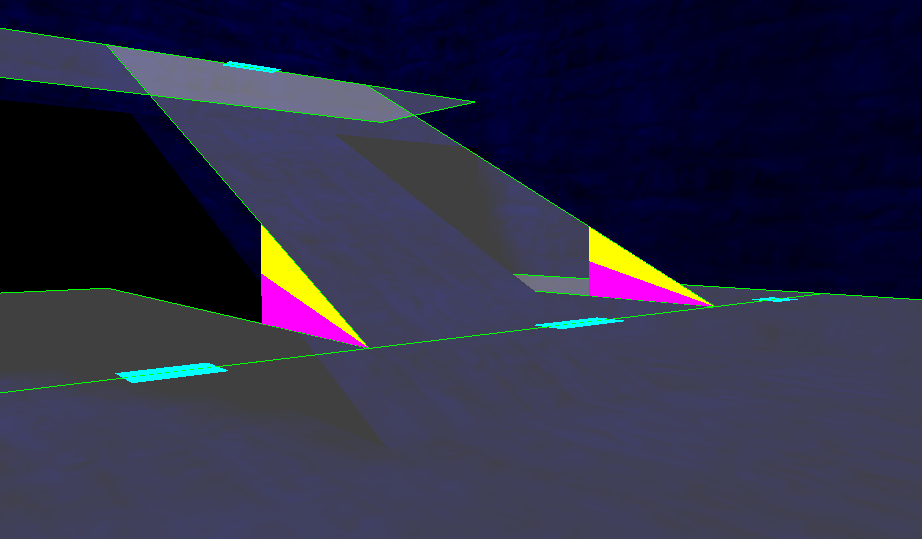(a) The navigation mesh for Ramp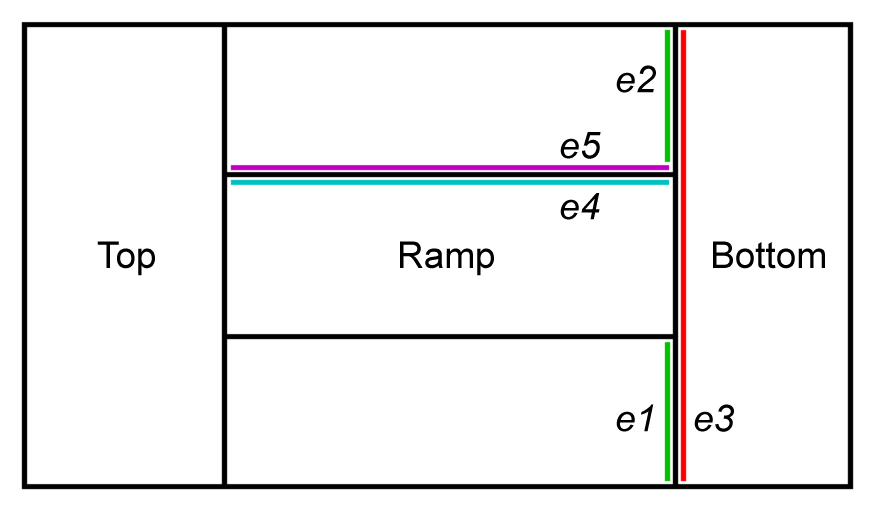(b) A top-down view, with some edges highlighted
Plane Same-Facing Edges Opposite-Facing Edges
1> {e1, 32} {e3}
2 {e4} {e5}
(c) The part of the edge plane table corresponding to the highlighted edges
Figure 4

A few details are needed to make this work:

• Vertical Plane Construction . Each edge is necessarily non-vertical (because it belongs to a walkable polygon), so the normal vector of a vertical plane through it can be calculated straightforwardly using the cross-product. If the endpoints of the edge are p1 and p2 , and û is once again the up vector, then the desired normal can be calculated as: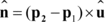Normalising this to give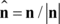, the equation of the desired plane is then: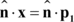• Canonical Plane Determination . Given a plane with equation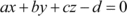, we define the ‘canonical’ form of this plane to be the one where the first of a , b or c to be non-zero is positive. Thus, the canonical form of both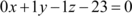and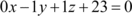would be. Note that a plane is either already in canonical form, or can be canonicalised straightforwardly by negating all of its coefficients.
• Plane Ordering . In order to use planes as the key for the edge plane table, we need to define a suitable way of ordering them. This can be done using a variant of the approach described in [ Salesin92 ]. In practice, the ordering was found to be easier to debug (although somewhat less efficient) if implemented as shown in Listing 3.
 ```function less: (lhs: Plane; rhs: Plane) -> bool var nL, nR: Vec3 := lhs.normal(), rhs.normal(); var dL, dR: double := lhs.dist(), rhs.dist(); // If these planes are nearly the same (in terms // of normal direction and distance value), then // neither plane is "less" than the other. var dotProd: double := nL.dot(nR); // acos(x) is only defined for x <= 1, so clamp // dotProd to avoid floating-point problems. if dotProd > 1.0 then dotProd := 1.0; var angle: double := acos(dotProd); var dist: double := dL - dR; if |angle| < εangle and |dist| < εdist then return false; var aL, bL, cL: double := nL.x, nL.y, nL.z; var aR, bR, cR: double := nR.x, nR.y, nR.z; // Otherwise, compare the two planes // "lexicographically". return (aL

To generate walk and step links, we transform each ordered pair 3 of opposing edges that lie in the same (canonical) plane into a 2D (orthonormal) coordinate system in the plane, and then determine the intervals (if any) in which links need to be created.

A suitable 2D coordinate system for a plane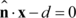can be generated as follows. To determine a suitable origin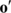for our coordinate system, we find the point on the plane that lies nearest to the world origin 0 : this is simply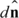. Given that the plane is vertical, suitable axis vectors for our coordinate system can be defined as: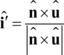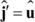This gives us a coordinate system in which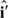is horizontal (in terms of the surrounding world) and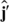is vertical. To transform an edge e on the plane into this new coordinate system, we transform each of its endpoints e1 and e2 as follows: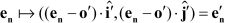Having transformed a pair of opposing edges e and f into the plane’s coordinate system, we next calculate the horizontal interval in which each edge lies; e.g. for e this would be: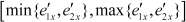If the horizontal intervals for the opposing edges do not overlap, then there can be no links between them. Otherwise, we compute the gradients m and cut points c of the lines y = mx + c through the two edges (still in the plane’s coordinate system) using basic mathematics; e.g. for e these would be: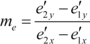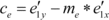Based on a comparison of the gradients, we then create the link segments in the plane as shown in Listing 4 and Figure 5.

 ```function calculate_link_segments : (e'1: Vec2; e'2: Vec2; f'1: Vec2; f'2: Vec2; xOverlap: Interval) -> LinkSegments xO = xOverlap; var result: LinkSegments; var me: double := (e’2y - e’1y) / (e’2x - e'1x); var mf: double := (f’2y - f’1y) / (f’2x - f'1x); var ce: double := e’1y - me * e’1x; var cf: double := f’1y - mf * f’1x; var Δm, Δc: double := mf - me, cf - ce; if |Δm| > ε then // If the gradients of the source and // destination edges are different, then we get // a combination of step up/step down links. // We want to find: // (a) The point walkX where yf = ye // (b) The point stepUpX where yf - ye = STEPTOL // (c) The point stepDownX where // ye - yf = STEPTOL var walkX: double := -Δc / Δm; var stepUpX: double := (STEPTOL - Δc) / Δm; var stepDownX: double := (-STEPTOL - Δc) / Δm; // Construct the step down and step up intervals // and clip them to the known x overlap interval. var sdI, suI: Interval := [min{walkX,stepDownX}, max{walkX,stepDownX}], [min{walkX,stepUpX}, max{walkX,stepUpX}]; sdI := intersect(sdI, xO); suI := intersect(suI, xO); // Construct the link segments. if not sdI.empty then result.downToF := [(sdI.low, me * sdI.low + ce), (sdI.high, me * sdI.high + ce)]; result.upToE := [(sdI.low, mf * sdI.low + cf), (sdI.high, mf * sdI.high + cf)]; if not suI.empty then <analogously> else if |Δc| < STEPTOL then // If the gradients of the source and destination // edges are the same (i.e. parallel edges), then // we either get a step up/step down combination, // or a walk link in either direction. var s1: Vec2 := (xO.low, me * xO.low + ce); var s2: Vec2 := (xO.high, me * xO.high + ce); var d1: Vec2 := (xO.low, mf * xO.low + cf); var d2: Vec2 := (xO.high, mf * xO.high + cf); if Δc > ε then // The destination is higher than the source. result.upToF := [s1,s2]; result.downToE := [d1,d2]; else if Δc < -ε then // The destination is lower than the source. result.downToF := [s1,s2]; result.upToE := [d1,d2]; else // The destination and source are level. result.walk := [s1,s2]; ``` Listing 4
 Creating links between edges: in (a), the gradients differ and a step down link is created from e to f in the region labelled sdI, and a step up link is created in the region labelled suI; in (b), the gradients are the same and a step up link is created from e to f in the region labelled xOverlap; in (c), a step down link is created from e to f in the region labelled xOverlap. The remaining case, of parallel edges leading to a walk link, is not shown. (a)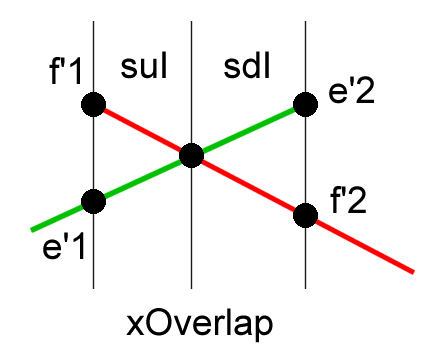(b)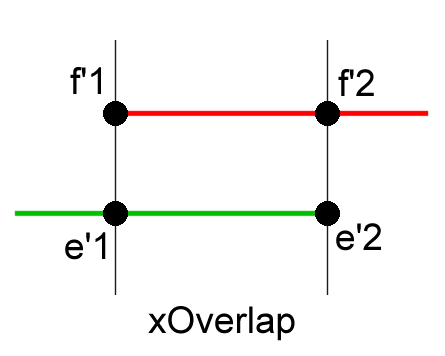(c)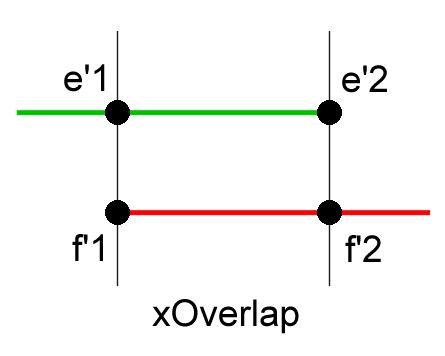Figure 5

The endpoints l 1 and l 2 of each link segment can be straightforwardly transformed back into world space to create the actual links as follows: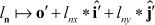## Potential extensions

One obvious extension is to add crouch links – these are links that tell agents when they need to crouch in order to traverse low areas (e.g. a low archway or a pipe). As the example in Figure 6 illustrates, these are inter-mesh links; they should be created so as to link the standing and crouching meshes for an agent at the boundary of areas that can be traversed on the crouching mesh but not on the standing one. Assuming that the AABBs for the two meshes differ only in their heights (as is the case in the example), one way of automatically detecting crouch links would be to match edges on the standing mesh that do not cause any walk or step links to be created with edges in the same plane on the crouching mesh that do.

 Creating crouch links between navigation meshes can allow tall characters to pass through low areas. Here, crouch links should be created between the standing (green) and crouching (red) meshes to allow agents to traverse this low archway. (c)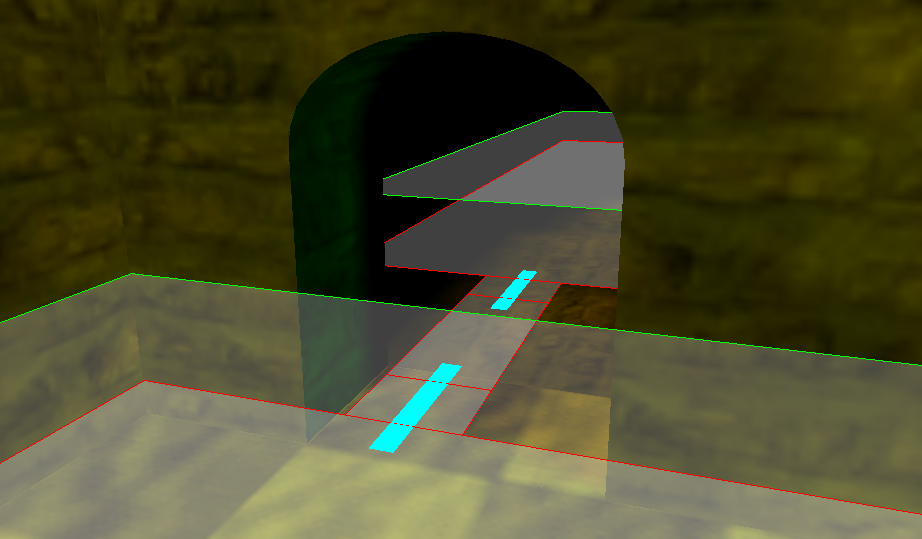Figure 6

## Conclusions

In this article, I have illustrated how to generate navigation meshes at an implementation level using an approach based on the work of van Waveren [ VanWaveren01 ]. Whilst there are many alternative techniques for navigation mesh construction, as surveyed in the introduction, this configuration space approach is useful because it allows us to avoid the difficulties regarding clearance height that have to be dealt with by other approaches; it also means that each agent occupies a single point on the mesh, completely avoiding the problems caused by an agent straddling multiple mesh polygons.

Navigation mesh generation , however, is only part of the picture – in a future article, I hope to write more about using navigation meshes for localisation, movement and path planning.

## Acknowledgements

As always, I would like to thank the Overload team for reviewing this article and suggesting ways in which to improve it.

## References

[Beucher90] Segmentation d’Images et Morphologie Mathématique (Image Segmentation and Mathematical Morphology). Serge Beucher. PhD thesis, E.N.S. des Mines de Paris, 1990.

[Gonzalez02] Digital Image Processing . Rafael C Gonzalez and Richard E Woods. Pearson Education, 2nd edition, 2002.

[Hale09] Full 3D ‘Spatial Decomposition for the Generation of Navigation Meshes’ D Hunter Hale and G Michael Youngblood. In Proceedings of the Fifth Artificial Intelligence for Interactive Digital Entertainment Conference , pages 142–147, 2009.

[Hamm08] ‘Navigation Mesh Generation: An Empirical Approach’ David Hamm. In Steve Rabin, editor, AI Game Programming Wisdom 4 , pages 113–123. Charles River Media, 2008.

[Hertel83] ‘Fast triangulation of simple polygons’ S Hertel and K Mehlhorn. In Proceedings of the 4th International Conference on the Foundations of Computation Theory , volume 158 of Lecture Notes in Computer Science, pages 207–218. Springer Verlag Berlin, 1983.

[hesperus] The hesperus 3D game engine. Stuart Golodetz. Source code available online at: https://github.com/sgolodetz/hesperus2 .

[Kallmann10] ‘Navigation Queries from Triangular Meshes’ Marcelo Kallmann. In Proceedings of the Third International Conference on Motion in Games (MIG) , pages 230–241. Springer-Verlag Berlin, 2010.

[Mononen09] Navigation Mesh Generation via Voxelization and Watershed Partitioning . Mikko Mononen. AiGameDev.com, March 2009. Slides available online (as of 30th July 2013) at https://sites.google.com/site/recastnavigation/MikkoMononen_RecastSlides.pdf .

[ORourke94] Computational Geometry in C , pages 60–61. Joseph O'Rourke. Cambridge University Press, 2nd edition, 1994.

[Pettré05] ‘A navigation graph for real-time crowd animation on multilayered and uneven terrain’ Julien Pettré, Jean-Paul Laumond and Daniel Thalmann. In Proceedings of the 1st International Workshop on Crowd Simulation , Lausanne, Switzerland, 2005.

[Ratcliff08] ‘Automatic Path Node Generation for Arbitrary 3D Environments’ John W Ratcliff. In Steve Rabin, editor, AI Game Programming Wisdom 4, pages 159–172. Charles River Media, 2008.

[Salesin92] Grouping Nearly Coplanar Polygons into Coplanar Sets. David Salesin and Filippo Tampieri. In David Kirk, editor, Graphics Gems III , pages 225–230. Morgan Kaufmann, 1992.

[Snook00] ‘Simplified 3D Movement and Pathfinding Using Navigation Meshes’ Greg Snook. In Mark DeLoura, editor, Game Programming Gems , pages 288–304. Charles River Media, 2000.

[Tozour02] ‘Building a Near-Optimal Navigation Mesh’ Paul Tozour. In Steve Rabin, editor, AI Game Programming Wisdom , pages 171–185. Charles River Media, 2002.

[Tozour04] ‘Search Space Representations’ Paul Tozour. In Steve Rabin, editor, AI Game Programming Wisdom 2 , pages 85–102. Charles River Media, 2004.

[VanToll11] ‘Navigation Meshes for Realistic Multi-Layered Environments’ Wouter G van Toll, Atlas F Cook IV and Roland Geraerts. In Proceedings of the IEEE/RSJ International Conference on Intelligent Robots and Systems (IROS) , pages 3526–3532, San Francisco, California, USA, 2011.

[VanToll12] ‘A navigation mesh for dynamic environments’ Wouter G van Toll, Atlas F Cook IV and Roland Geraerts. Computer Animation and Virtual Worlds , 23:535–546, 2012.

[VanWaveren01] The Quake III Arena Bot . Jean Paul van Waveren. Master’s thesis, Delft University of Technology, 2001.

1. A normal to a polygon is a vector that is perpendicular to the plane containing the polygon. In practice, since polygons are often oriented (i.e. they are considered to have a front face and a back face), it is common for implementations to calculate normals that point out of the front faces of polygons. It is also sometimes convenient for implementations to normalise the calculated normals (that is, to make them unit length), in which case normals for polygons are uniquely defined and we can talk about the normal rather than a normal to a given polygon.
2. A voxel (or volume element ) is the 3D equivalent of a pixel, so voxelizing a 3D environment means converting from e.g. a polygonal representation of the environment to a representation consisting of many small cubes.
3. Note that because the pairs are ordered, we consider each unordered pair of edges twice when creating links, once in each direction.